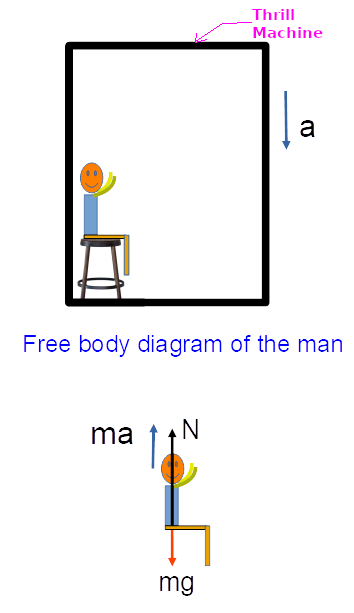# In thrill machine rides at amusement parks , there can be an acceleration of 3g or more . But...

## Question:

In thrill machine rides at amusement parks , there can be an acceleration of 3g or more . But without head rests , acceleration like this would not be safe. Think why not?

## Pseudo Force:

When the motion of an object is described using a non-inertial reference frame (a frame having non-zero acceleration) then, an apparent force acts on the object. This apparent force is known as Pseudo force.

It is also called as fictitious force, inertial force or d'Alembert force.

Let:

• The acceleration of the thrill machine is {eq}a {/eq} along the downward direction.

The free-body diagram of the person is shown in the figure below.In the above figure,

• a is the acceleration of the thrill machine.
• mg is the weight of the person.
• N is the normal reaction force on the person.
• ma is the pseudo force on the person when observed by an observer sitting on the thrill machine.

Here,

{eq}\begin{align} N &= mg-ma\\ &= mg-m(3g)\\ &= -2mg\\ \end{align} {/eq}

Since we got the normal reaction force on the person is negative that means the person start going up with respect to the thrill machine. So, we must need a head rests for a safe journey.